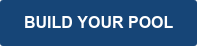When choosing your inground liner, you will need to know how to convert Decimals(Feet) to Inches.

In this blog we will explain how to convert a measurement that is in decimal (feet) to inches or even down to the eighth of an inch.  Don't know what decimal (feet) is? Have you ever seen a measurement in feet yet it is not a whole number?  For example, 4.62'.  This is a measurement in decimal (feet). Sometimes you need to know what the .62 part of the measurement is.  That is what this blog is for, how to convert that decimal amount into inches, even down to an eighth of an inch.

As a quick reference before we start, most measurements required when measuring for a vinyl swimming pool liner should be rounded to the nearest inch. In some cases, however, you may want or need the measurement to be more precise.

Here is a quick and simple reference on converting inches to feet and rounding to the nearest inch. This is a great tool when you need to calculate square feet or add measurements together using a calculator.

1" …. .08         2" …. .17         3" …. .25         4" …. .33         5" …. .42         6" …. .50

7" …. .58         8" …. .67         9" …. .75         10" …. .83       11" …. .92       12" …. 1.0

This can also be done by multiplying the inches times .08333 (1/12th), this will give you the exact number, not the rounded number. This can also be very helpful if you have a measurement in inches and want to quickly convert to feet.  For example, you have a pool that you measured the total width as 150" you can multiply 150 x .08333 which equals 12.4995 or 12'-6".

Now that you understand how to convert inches to feet, here's a chart and we'll further explain how to calculate down to the eighth of an inch. In the chart below, the math is done for you to make this as easy as possible. If you are like most of us, you want to better understand the math involved in doing this calculation, so we explain the process and provide an example below the chart.Now that you have reviewed the conversion chart, here's an example.

Let's assume you have a measurement in decimal form which equals 33.5528' and want to convert it to Feet and Inches instead of just Feet. By looking at the chart above we know 33.5528' is 33' 6-5/8", but how do we get this conversion. It is done using a two-step process, see below…

• Step 1: Take the numbers to the right of the decimal and divide by .08333 (1/12th). This will give you the whole inches to the left of the decimal and the amount less than an inch to the right. For this example, you will take 0.5528 of 33.5528' and divide by .08333 which will equal 6.6339. So now we have 33' - 6.6339"
• Step 2: To determine what the eighths of an inch will be, simply divide the amount from right of the decimal by .125 and round the result to the nearest whole number. This gives you the top of the eighth fraction. From our example, this would be .6339/.125 = 5.0712. Round down to 5. So the fraction will be 5/8".

Our final result for this conversion is 33' 6-5/8"

### THERE'S AN APP FOR THAT

The photo below was taken from a free iPhone app named "Decimals and Fractions by Grand Unified, LLC."  This app is a very simple program that will quickly convert a fraction to decimal or decimal to fraction and even shows the remaining amount in plus or minus form. The program will also reduce the fraction. As shown in the photo below, we used the app to calculate step 2.  So, whether you prefer using the chart above, a standard calculator, or an iPhone app you should be able to convert a decimal amount to inches and to an eighth of an inch.## If you are now ready to order your liner, you will need some of these resources!

Measurement and Quote Form 1 is required for all pool shapes and sizes. Click the link below to download Form 1.

Measurement and Quote Form 2 is required if you are ordering a liner for an L-shape pool or if your pool has Vinyl Covered Steps. NOTE: Form 1 must also be completed and attached to Form 2. Click the link below to download Form 2.

If you are unsure of how to measure your pool, please view our detailed measuring instructions or have a pool professional measure your pool to ensure correct and complete measurements are recorded. Click the link below to download Liner Measuring Instructions.

Freeform pools such as a Kidney or Deer Creek pool are very common but require more measurements than a rectangle pool. To ensure we create a liner that fits properly for your freeform pool, we require you to complete an A-B Point to Point Measurement Form. We have made instructions available so that you can complete the form yourself. Click the links below to download the A-B Point to Point Measurement Form and Instructions.

### Filed Under:

Tips, How-To, Inground Pools, Liners